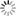# Episode List

OR

### Season 2

1 Jul. 1955
Diplomatic ErrorBe the first one to add a plot.

8 Jul. 1955
Strangle HoldBe the first one to add a plot.

15 Jul. 1955
The Corpse in Room ThirteenBe the first one to add a plot.

22 Jul. 1955
Death Walks by NightBe the first one to add a plot.

29 Jul. 1955
The VerdictBe the first one to add a plot.

5 Aug. 1955
The BargainBe the first one to add a plot.

19 Aug. 1955
Death in WhiteBe the first one to add a plot.

26 Aug. 1955Be the first one to add a plot.

2 Sep. 1955
Never Let Me DieBe the first one to add a plot.

9 Sep. 1955
Bond of HateAn ever- quarreling, long married couple take a holiday in the mountains, where the wife seeks to take revenge on her husband, whom she believes cut short her concert career.

16 Sep. 1955Be the first one to add a plot.

23 Sep. 1955
Side EntranceBe the first one to add a plot.

30 Sep. 1955
The Search for Martha HarrisBe the first one to add a plot.

7 Oct. 1955
Money to BurnBe the first one to add a plot.

14 Oct. 1955
Two of a KindBe the first one to add a plot.

21 Oct. 1955
Cross ChannelBe the first one to add a plot.

28 Oct. 1955
Death Takes No HolidayBe the first one to add a plot.

4 Nov. 1955Be the first one to add a plot.

11 Nov. 1955
Stranger in TownBe the first one to add a plot.

18 Nov. 1955
By Persons UnknownBe the first one to add a plot.

25 Nov. 1955
Killer at LargeBe the first one to add a plot.

2 Dec. 1955
Wrong Time MurderBe the first one to add a plot.

9 Dec. 1955
8.7 (6)Bill Kent stumbles on a package of diamonds from an air wreckage and tries to sell them illegally through his wife's lover.

16 Dec. 1955
Second SightBe the first one to add a plot.

23 Dec. 1955
Code Name: MurderBe the first one to add a plot.

30 Dec. 1955
Snapshot